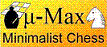## Wu / Beal retrograde analisys algorithm

Programming Topics (Computer Chess) and technical aspects as test techniques, book building, program tuning etc

Moderator: Andres Valverde

### Wu / Beal retrograde analisys algorithm

Hi everyone,
I would like to implement this memory-efficient retrograde analisys algorithm witch uses one bit in ram for every position.
Does anyone have or knows source code for this one?

Can someone explain the ProveSucessor() function in the pseudo code below?

best regards,
Alvaro

Code: Select all
`DATABASE W, B; // full databases (i.e. depth to win/loss for each position),// W for White-to-move positions, B for Black-to-move,// sequential access onlySBITS S; // sequential access only bitmapRBITS R; // random access bitmapvoid TopLevel{   DoInitialize();   n = 0; // depth to mate or conversion   while (!DoneWhite() && !DoneBlack())   {      if (!DoneWhite()) // last pass added new positions      {         S = Load(W, WIN_IN_N(n)); // S = WTM win_in_n         R = Predecessor(S); // R = BTM predecessors of S         S = Load(B, UNKNOWN); // S = BTM unknown         S = S & R; // S = BTM maylose_in_n         R = Load(W, WIN_<=_N(n)); // R = WTM win_in_n or less         S = ProveSuccessor(S, R); // S = BTM lose_in_n         B = Add(S, LOSE_IN_N(n)); // B += S         if (dtm) // distance_to_mate?            S = Load(B, LOSE_IN_N(n)); // S = BTM lose_in_n         R = Predecessor(S); // R = WTM maybe win_in_n+1         S = Load(W, UNKNOWN); // S = WTM unknown         S = S & R; // S = WTM win_in_n+1         W = Add(S, WIN_IN_N(n+1)); // W += S      }      if (!DoneBlack()) // done for BTM?      {         S = Load(B, WIN_IN_N(n)); // S = BTM win_in_n         R = Predecessor(S); // R = WTM predecessors of S         S = Load(W, UNKNOWN); // S = WTM unknown         S = S & R; // S = WTM maylose_in_n         R = Load(B, WIN_<=_N(n)); // R = BTM win_in_n or less         S = ProveSuccessor(S, R); // S = WTM lose_in_n         W = Add(S, LOSE_IN_N(n)); // W += S         if (dtm) // distance_to_mate?            S = Load(W, LOSE_IN_N(n)); // S = WTM lose_in_n         R = Predecessor(S); // R = BTM maybe win_in_n+1         S = Load(B, UNKNOWN); // S = BTM unknown         S = S & R; // S = BTM win_in_n+1         B = Add(S, WIN_IN_N(n+1)); // B += S      }      n = n + 1;  }}`
Alvaro Jose Povoa Cardoso

Posts: 2
Joined: 10 Oct 2005, 22:56

### Re: Wu / Beal retrograde analisys algorithm

I am not sure how this algorithm could ever compete with the straightforward
Code: Select all
`do  cnt=0;  for all positions X    if label[X] == n      for all white (retro-)moves X->X´        if mark[X´] == 0          mark[X´] = 1;          for all black (retro-)moves X´->X"            LABEL_GRANDMOTHERwhile cnt != 0LABEL_GRANDMOTHER:  if label[X"] == UNDECIDED    label[X"] = n+1; cnt++;    for all black moves X"->Y      if mark[Y] == 0        label[X"] = UNDECIDED; cnt--;        break;`

With the TB organized such that the black pieces scan fastest, all accesses after black moves will typically be cache hits. If some of the moves of the white pieces would be so far away in the TB that they would result in cache misses, You could trivially split the retrograde tree at the root, treating the moves of those white pieces in a TB scan with the X loop scanning those pieces with reversed nesting.

By packing label[] and mark[] in a single byte (i.e. label[X] is TB[X]>>1 and mark[X] is TB[X]&1) this uses only a single byte per position. This means that 5-men TB easily fit in memory. (And some 6-men TB as well, on bigger computers). In that case you can even speed it up more by doing pre-counting of the black escape moves, so that the screening of the potentially lost in n+1 states can be done very quickly:
Code: Select all
`LABEL_GRANDMOTHER:  if label[X"] > n+1    label[X"]++;    if label[X"]==0      label[X"]=n+1; cnt++;`

This would require the pre-counting
Code: Select all
`for all positions X  for all black moves X->X´    if mark[X´]==0      label[X]--;`

(For storing on disk this is probably not recommended, as it makes the data difficult to compress.)H.G.Muller

Posts: 3222
Joined: 16 Nov 2005, 12:02
Location: Diemen, NL

### Re: Wu / Beal retrograde analisys algorithm

Alvaro Jose Povoa Cardoso wrote:Can someone explain the ProveSucessor() function in the pseudo code below?

To answer your question, btw, the routine ProveSuccessor(S, R) scans for set bits in S (corresponding to the 'may lose' positions, that are a predecessor of a position that was just resolved as won for the opponent, and were not yet resolved themselves), and tries all moves of the STM from that position. For the positions these moves lead to, it then checks (in the bit set R) if they all lead to positions that are won for the opponent in n or less.

If it is, the bit in S remains set. If there is a single move that leads to a position that is not (yet) won for the opponent (the corresponding bit in R is clear), then it resets the bit in S. So you can stop generating moves after the first 'escape' for the STM that you find.H.G.Muller

Posts: 3222
Joined: 16 Nov 2005, 12:02
Location: Diemen, NL